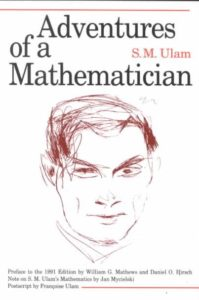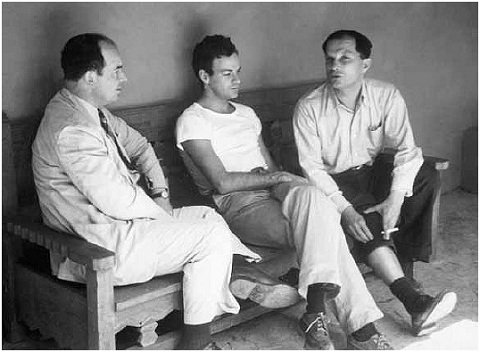I have read recently again the auto-biography of Ulam entitled Adventures of a Mathematician. Stanislaw Marcin Ulam (1909 – 1984) is a famous Polish – American mathematician, just like Mark Kac (1914 – 1984). Ulam and Kac come roughly from the same part of Poland, were interested by fundamental and applied mathematics, probability, and physics, moved from Poland to the United States of America, lived during the same period, and wrote an interesting auto-biography. Both died in 1984. Here is an excerpt from pages 269 – 270:

Mark Kac had also studied in Lwów, but since he was several years younger than I (and I had left when only twenty ­six myself), I knew him then only slightly. He told me that as a young student he had been present at my doctorate ceremony and had been impressed by it. He added that these first impressions usually stay, and that he still considers me “a very senior and advanced person,” even though the ratio of our ages is now very close to one. He came to America two or three years after I did. I remembered him in Poland as very slim and slight, but here he became rather rotund. I asked him, a couple of years after his arrival, how it had happened. With his characteristic good humor he replied: “Prosperity!” His ready wit and almost constant joviality make him extremely congenial.

After the war he visited Los Alamos, and we developed our scientific collaboration and friendship. After a number of years as a professor at Cornell he became a professor of mathematics at The Rockefeller Institute in New York (now The Rockefeller University.) He and the physicist George Uhlenbeck have established mathematics and physics groups at this Institute, where biological studies were the principal and almost exclusive subject before.

Mark is one of the very few mathematicians who possess a tremendous sense of what the real applications of pure mathematics are and can be; in this respect he is comparable to von Neumann. He was one of Steinhaus‘s best students. As an undergraduate he collaborated with him on applications of Fourier series and transform techniques to probability theory. They published several joint papers on the ideas of “independent functions.” Along with Antoni Zygmund he is a great exponent and true master in this field. His work in the United States is prolific. It includes interesting results on probability methods in number theory. In a way, Kac, with his superior common sense, as a mathematician is comparable to Weisskopf and Gamow as physicists in their ability to select topics of scientific research which lie at the heart of the matter and are at the same time of conceptual simplicity. In addition — and this is perhaps related — they have the ability to present to a wider scientific audience the most recent and modern results and techniques in an understandable and often very exciting manner. Kac is a wonderful lecturer, clear, intelligent, full of sense and avoidance of trivia.

Among the mathematicians of my generation who influenced me the most in my youth were Mazur and Borsuk. Mazur I have described earlier. As for Borsuk, he represented for me the essence of geometrical intuition and truly meaningful topology. I gleaned from him, without being able to practice it myself, the workings of n­ dimensional imagination. Today Borsuk is continuing his creative work in Warsaw. …

Ulam’s auto-biography contains information and anecdotes on the personalities of great scientists of the twentieth century, such as Stefan Banach, Hugo Steinhaus, and Stanisław Mazur, from Lwów, but also Kazimierz Kuratowski, from Warsaw, and later on Paul Erdős, George David Birkhoff, John von Neumann, Richard Feynman, Niels Bohr, Enrico Fermi, and George Gamow. Many of the last ones were involved, like Ulam, in the Manhattan project in Los Alamos.

Ulam was an open, deep, and creative mind, interested by all aspects of applied and fundamental mathematics, and beyond! In Los Alamos, Ulam invented, in collaboration with von Neumann, the Monte-Carlo method and also particle numerical methods in fluid mechanics. He moreover discovered chaos in non-linear vibrations with Fermi and Pasta. Last but not least, Ulam played an essential role in the design of the hydrogen nuclear bomb together with Edward Teller. Ulam’s work on nuclear chain reactions led him to the study of what we call now branching processes, and the discovery of the generating function method. Here is below an excerpt taken from pages 159-160. [I forgot to mention this interesting historical fact at the end of the chapter on branching processes in my book Recueil de modèles aléatoires with my old friend Florent Malrieu – A shame!]

We discussed problems of neutron chain reactions and the probability problems of branching processes, or multiplicative processes, as we called them in 1944.

I was interested in the purely stylized problem of a branching tree of progeny from one neutron which may multiply, into zero (that is, the death of a neutron by absorption), or one (that just continues itself), or two or three or four (that is, causes the emergence of new neutrons), each possibility with a given probability. The problem is to follow the future course and the chain of possibilities through many generations.

Very early Hawkins and I detected a fundamental trick to help study such branching chains mathematically. The so­ called characteristic function, a device invented by Laplace and useful for normal “addition” of random variables, turned out to be just the thing to study “multiplicative” processes. Later we found that observations to this effect had been made before us by the statistician Lotka, but the real theory of such processes, based on the operation of iteration of a function or of operators allied to the function (a more general process), was begun by us in Los Alamos, starting with a short report. This work was strongly generalized and broadened in 1947, after the war, by Everett and myself after he joined me in Los Alamos. Some time later, Eugene Wigner brought up a question of priorities. He was eager to note that we did this work quite a bit before the celebrated mathematician Andrei N. Kolmogoroff and other Russians and some Czechs had laid claim to having obtained similar results.

In modern terms, if $Z_n$ is the number of neutrons at generation $n$, with $Z_0:=1$, then the branching process ${(Z_n)}_{n\geq0}$ modeling the neutron chain reaction can be written as $$Z_{n+1}=\sum_{k=1}^{Z_n}X_{n+1,k}$$ where ${(X_{n,k})}_{n,k\geq1}$ are independent and identically distributed random variables with offspring distribution $P:=p_0\delta_0+p_1\delta_1+\cdots$. For any discrete random variable $X$, we denote by $$g_X(s):=\mathbb{E}(s^X)=\sum_{k=0}^\infty\mathbb{P}(X=k)s^k$$ its generating function at point $s\in[0,1]$. We have then, with $X\sim P$ and $g:=g_X$, $$g_{Z_{n+1}}(s)=\mathbb{E}(\mathbb{E}(s^{X_1+\cdots+X_{Z_n}})\mid Z_n))=\mathbb{E}(\mathbb{E}(s^X)^{Z_n})=g_{Z_n}(g_X(s))=\cdots=g^{\circ (n+1)}(s).$$ It remains to use fixed point analysis to get the behavior of the extinction probability $$\mathbb{P}(\exists n:Z_n=0)=\lim_{n\to\infty}\mathbb{P}(Z_n=0)=\lim_{n\to\infty}g^{\circ n}(0).$$

But you may prefer the aristocratic British families of Francis Galton and Henry William Watson.John von Neumann, Richard Feynman, and Stan Ulam on the porch of Bandelier lodge in Frijoles Canyon, New Mexico, during a picnic, ca 1949 (Nicholas Metropolis). Both photo and legend appear in Ulam’s auto-biography.